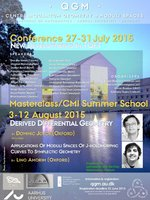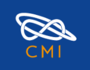# Centre for Quantum Geometry of Moduli Spaces# Masterclass/CMI Summer School Aug 2015

Derived Differential Geometry by Dominic Joyce & Applications of moduli spaces of J-holomorphic curves to symplectic geometry by Lino Amorim

2014.12.11 | Jane Jamshidi

 Date Mon 03 Aug — Wed 12 Aug Time 09:00  —  16:00 Location Aud.D3 (1531-215), Department of Mathematics
Registration has closed

Derived Differential Geometry
by Dominic JoyceAbstract: Derived Differential Geometry is the study of derived smooth manifolds and orbifolds, where "derived" is in the sense of the Derived Algebraic Geometry of Jacob Lurie  and Toën-Vezzosi [14,15]. There are by now several different models for (higher) categories of derived manifolds and derived orbifolds: the "derived manifolds" of Spivak , the "d-manifolds" and "d-orbifolds" of Joyce [9,10], the "derived manifolds" of Borisov-Noel [1,2], and the "M-Kuranishi spaces" and "Kuranishi spaces" of Joyce . For derived manifolds without boundary, these are all known to be roughly equivalent, at least at the level of homotopy categories.

Actually, a prototype version of derived orbifolds has been used for many years in the work of Fukaya, Oh, Ohta and Ono [4,5,6] as their "Kuranishi spaces", a geometric structure on moduli spaces of J-holomorphic curves in symplectic geometry, but it was not understood until recently that these are part of the world of derived geometry - see .

Derived manifolds are a (higher) category of geometric spaces which include ordinary smooth manifolds, but also many more singular objects - for instance, if X,Y are embedded submanifolds of a manifold Z, then the intersection X n Y has the structure of a derived manifold of dimension dim X + dim Y - dim Z (which can be negative), and any closed subset S of Rcan be given the structure of a derived manifold of dimension n - 1. They have a "derived" geometric structure, which is difficult to define, but means that derived manifolds have their own differential geometry, and in many ways behave as well (and sometimes better) than smooth manifolds.

One reason derived manifolds and derived orbifolds are important is that many moduli spaces (families of isomorphism classes geometric objects) in differential geometry, and in complex algebraic geometry, can be given the structure of derived manifolds and derived orbifolds.  For example, any moduli space of solutions of a nonlinear elliptic partial differential equation on a compact manifold is a derived manifold. Also, compact, oriented derived manifolds and derived orbifolds have "virtual classes", generalizing the fact that a compact, oriented n-dimensional manifold X has a fundamental class [X] in its top-dimensional homology group Hn(X,Z). This means that derived manifolds and orbifolds have applications in enumerative invariant problems (e.g. Donaldson invariants, Gromov-Witten invariants, Seiberg-Witten invariants, Donaldson-Thomas invariants, ...), and generalizations such as Floer homology theories and Fukaya categories.

This lecture course will define and discuss 2-categories of derived manifolds and derived orbifolds, and their applications to moduli spaces of solutions of nonlinear elliptic partial differential equations including J-holomorphic curves, and "counting" problems in differential geometry, and complex algebraic geometry. We explain two different ways to define these 2-categories, firstly using C-rings, C-schemes and C-algebraic geometry [7,8], following Spivak , Joyce [9,10] and Borisov-Noel [1,2], or secondly using an "atlas of charts" (Kuranishi neighbourhoods) approach in , which is based on Fukaya et al. [4,5,6]. (See reading list below)

Applications of moduli spaces of J-holomorphic curves to symplectic geometry
by Lino Amorim

Abstract: The main goal of this course is to show how the moduli spaces of J-holomorphic curves can be used to associate to each Lagrangian submanifold an A-infinity algebra on its cohomology, following the work of Fukaya-Oh-Ohta-Ono.
We will start with a review of some basic concepts in symplectic geometry and an overview of the main questions in Lagrangian intersection theory. Then we introduce the moduli space of J-holomorphic curves and define Lagrangian Floer cohomology in Floer's original setting.
Next we analyze the difficulties encountered in trying to extend the definition of Floer cohomology to more general contexts. These lead us to consider A-infinity algebras. At this point we make a small digression into the homological algebra of A-infinity algebras, bimodules and categories.
We reach the heart of the course where we explain how by making the moduli spaces of J-holomorphic disks with boundary in a Lagrangian submanifold into Kuranishi spaces we can construct an A-infinity algebra on the cohomology of the Lagrangian. Additionally we define an A-infinity bimodule for each pair of Lagrangians.
Finally we organize all the Lagrangians for which Floer cohomology can be defined into an A-infinity category known as the Fukaya category of the ambient symplectic manifold. We end by discussing the Fukaya category's role in Kontsevich's Homological Mirror Symmetry Conjecture.

Social programme

• Monday 3/8 at 6pm: Social networking incl. light supper. Free of charge.
• Tuesday 11/8 at 6pm: Special dinner at a restaurant in town. This costs DKK 375 (three course meal incl. a wine menu). Registration deadline Thursday 6 August 2015 - register at: auws.au.dk/default.aspx

Included in the event

• Morning and afternoon coffee/tea
• Lunches on weekdays
• Social dinner (first night)

In special cases, QGM is able to offer support for accommodation to junior researchers (PhD students and postdocs). Only applications received before 8 June 2015 will be considered.

OrganizersJørgen Ellegaard Andersen (QGM, AU)
Niels Leth Gammelgaard (QGM, AU)

Organized in partnership with the Clay Mathematics Institute

Participants
Alboresi, Davide (Utrecht University)
Amorim, Joana (Oxford Brookes University)
Bahayou, Amine (Kasdi Merbah University)
Bjerre, Mette (QGM, Aarhus University)
Bischoff, Francis  (University of Toronto)
Branco, Lucas (University of Oxford)
Caputo, Raffaele (University of Hamburg)
Cotti, Giordano (SISSA)
Chan, Joseph (University of Melbourne)
Dvirnas, Albertas (Lund University)
Ens, Travis (University of Toronto)
Huang, Yang (QGM, Aarhus University)
Joonhyung, Kim (Hannam University)
Kanstrup, Tina (QGM/Aarhus University)
Kozhasov, Khazhgali (SISSA)
Marzioni, Simone, (QGM, Aarhus University)
Malusà, Alessandro (QGM, Aarhus University)
Matviichuk, Mykola  (University of Toronto)
Rasmussen, Kenneth (QGM, Aarhus University)
Rembardo, Gabriele (Paris VI)
Ungheretti, Massimiliano (University of Copenhagen)
Vo, Huan (University of Toronto)
Wilkins, Nicholas (University of Oxford)
Zerouali, Ahmed (University of Toronto)

Note: A lot of these references are rather difficult to read for the uninitiated - the point of the lectures is to explain them. The most accessible are probably the survey papers , , , , plus , which needs only background in differential geometry.

 D. Borisov, 'Derived manifolds and Kuranishi models', arXiv:1212.1153.
 D. Borisov and J. Noel, 'Simplicial approach to derived differential manifolds', arXiv:1112.0033.
 E.J. Dubuc, 'C-schemes', Amer. J. Math. 103 (1981), 683-690.
 K. Fukaya, 'Floer homology of Lagrangian submanifolds', arXiv:1106.4882.
 K. Fukaya, Y.-G. Oh, H. Ohta and K. Ono, 'Lagrangian intersection Floer theory - anomaly and obstruction', Parts I and II. A.M.S./International Press, 2009.
 K. Fukaya and K. Ono, 'Arnold Conjecture and Gromov-Witten invariant', Topology 38 (1999), 933-1048.
 D. Joyce, 'Algebraic Geometry over C-rings', arXiv:1001.0023, 2010.
 D. Joyce, 'An introduction to C-schemes and C-algebraic geometry', arXiv:1104.4951.
 D. Joyce, 'An introduction to d-manifolds and derived differential geometry', pages 230-281 in L. Brambila-Paz et al. 'Moduli spaces', L.M.S. Lecture Notes 411, C.U.P., 2014. arXiv:1206.4207.
 D. Joyce, 'D-manifolds, d-orbifolds and derived differential geometry: a detailed summary'arXiv:1208.4948.
 D. Joyce, 'A new definition of Kuranishi space', arXiv:1409.6908
 J. Lurie, 'Derived Algebraic Geometry V: Structured spaces', arXiv:0905.0459.
 D.I. Spivak, 'Derived smooth manifolds', Duke Mathematical Journal 153 (2010), 55-128. arXiv:0810.5174.
 B. Toën, 'Derived Algebraic Geometry', arXiv:1401.1044.
 B. Toën and G. Vezzosi, 'Homotopical Algebraic Geometry II: Geometric Stacks and Applications', Mem. Amer. Math. Soc. 193 (2008), no. 902. math.AG/0404373.

Public/media